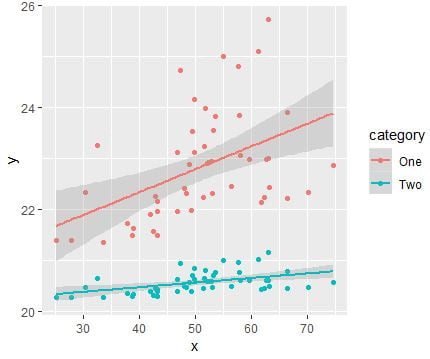[This article was first published on R tutorials – Statistical Aid: A School of Statistics, and kindly contributed to R-bloggers]. (You can report issue about the content on this page here)
Want to share your content on R-bloggers? click here if you have a blog, or here if you don't.

The Pearson correlation coefficient, sometimes known as Pearson’s r, is a statistic that determines how closely two variables are related. Its value ranges from -1 to +1, with 0 denoting no linear correlation, -1 denoting a perfect negative linear correlation, and +1 denoting a perfect positive linear correlation. A correlation between variables means that as one variable’s value changes, the other tends to change in the same way.

## Creating or Importing data into R

Let’s import data into R or create some example data as follows:

```set.seed(150)
data <- data.frame(x = rnorm(50, mean = 50, sd = 10),
random = sample(c(-10:10), 50, replace = TRUE))
data\$y <- data\$x + data\$random```

If we want to calculate the Pearson’s correlation of x and y in data, we can use the following code:

```correlation <- cor(data\$x, data\$y, method = 'pearson')
Checking the results: > correlation
 0.9025428```

From the above result, we get that Pearson’s correlation coefficient is 0.90, which indicates a strong correlation between x and y.

### Interpretation of Pearson Correlation Coefficient

The value of the correlation coefficient (r) lies between -1 to +1. When the value of –

• r=0; there is no relation between the variable.
• r=+1; perfectly positively correlated.
• r=-1; perfectly negatively correlated.
• r= 0 to 0.30; negligible correlation.
• r=0.30 to 0.50; moderate correlation.
• r=0.50 to 1 highly correlated.

A common misconception about the Pearson correlation is that it provides information on the slope of the relationship between the two variables being tested. This is incorrect, the Pearson correlation only measures the strength of the relationship between the two variables. To illustrate this, consider the following example:

```set.seed(150)
xvalues <- rnorm(50, mean = 50, sd = 10)
random <- sample(c(10:30), 50, replace = TRUE)
data <- data.frame(x = rep(xvalues, 2),
random = rep(random, 2),
category = rep(c("One","Two"), each = 50))
data\$y[data\$category=="One"] <- 20 + data\$x[data\$category=="One"]/data\$random[data\$category=="One"]
data\$y[data\$category=="Two"] <- 20 + data\$x[data\$category=="Two"]/(5*data\$random[data\$category=="Two"])
correlation.one <- cor(data\$x[data\$category=="One"], data\$y[data\$category=="One"], method = 'pearson')
correlation.two <- cor(data\$x[data\$category=="Two"], data\$y[data\$category=="Two"], method = 'pearson')```

The Pearson correlation coefficient of these two sets of x and y values is exactly the same:

```> correlation.one
 0.462251
> correlation.two
 0.462251```

However, when we plot these x and y values on a chart, the relationship looks very different:

```library(ggplot2)
gg <- ggplot(data, aes(x, y, colour = category))
gg <- gg + geom_point()
gg <- gg + geom_smooth(alpha=0.3, method="lm")
print(gg)```Learn Data Science and Machine Learning

Data Analysis Using R/R Studio

The post Pearson correlation in R appeared first on Statistical Aid: A School of Statistics.